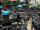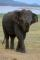Guess number

With what number should be divided number 9727 to be reduced by 70%?

Result

x =  3.3

Solution:Leave us a comment of example and its solution (i.e. if it is still somewhat unclear...):Be the first to comment!To solve this example are needed these knowledge from mathematics:

Our percentage calculator will help you quickly calculate various typical tasks with percentages. Do you have a linear equation or system of equations and looking for its solution? Or do you have quadratic equation?

Next similar examples:

1. Dropped sheetsThree consecutive sheets dropped from the book. The sum of the numbers on the pages of the dropped sheets is 273. What number has the last page of the dropped sheets?
2. Unknown number 11That number increased by three equals three times itself?
3. Find xSolve: if 2(x-1)=14, then x= (solve an equation with one unknown)
4. The quotientThe quotient of g and 55 is the same as 279. What is g?
5. ClassIn 7.C clss are 10 girls and 20 boys. Yesterday was missing 20% of girls and 50% boys. What percentage of students missing?
6. Volatile percentsKent is working as a forex trader. Suffered a loss of 65.8 percent. Calculate what percentage must now earn to lift from loss?
7. Negative in equation2x + 3 + 7x = – 24, what is the value of x?
8. 100 %How much is 100%, if 17 % is 1169?
9. Simple equation 2Find X in this simple equation: X/9 = 96/108
10. IronIron ore contains 57% iron. How much ore is needed to produce 20 tons of iron?
11. Seeds 2How many seeds germinated from 1000 pcs, when 23% no emergence?
12. Forest nurseryIn the forest nursery after winter, they found that 1/10 stems died out of them. For them, they land 193 new spruces. How many spruces are in the forest nursery?
13. Equation 29Solve next equation: 2 ( 2x + 3 ) = 8 ( 1 - x) -5 ( x -2 )
14. Simple equationSolve for x: 3(x + 2) = x - 18
15. Simple equationSolve the following simple equation: 2. (4x + 3) = 2-5. (1-x)
16. NormThree workers planted 3555 seedlings of tomatoes in one dey. First worked at the standard norm, the second planted 120 seedlings more and the third 135 seedlings more than the first worker. How many seedlings were standard norm?
17. EquationSolve equation and check the result: 1.4x - 3/2 + x - 9,8 = x + 0,4/3 - 7 + 1,6/6